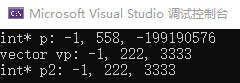## Opencv 基本操作四 指针数组、vector与Mat之间的相互转换 | Mat切片成Vector＜mat＞并还原_vector mat 相互转化-程序员宅基地

#### 1、指针数组与vector之间的相互转换

##### 指针转vector

std::vector vp(p, p + 1000);，其中p为指针的名称，1000为指针的数据长度

##### vector转指针

int* p2 = vp.data();//浅拷贝（p2与vp共用同一片内存区域）

//重载vector的运算符
template <typename T>
vector<T>& operator +(vector<T>& v1, vector<T>& v2)
{

v1.insert(v1.end(), v2.begin(), v2.end());
return v1;
}

//指针数组转vector
int* p = new int;//空间分配方式一
p = (int*)malloc(1000*sizeof(p));//空间分配方式二：malloc赋值方式
p = -1;

std::vector<int> vp(p, p + 1000);//深拷贝（把起始地址到结束地址的值拷贝一遍）
vp = 222;
//-------实现vector的拼接--------
std::vector<int> vp2 = vp + vp;
//vecort转指针数组
int* p2 = vp.data();//浅拷贝（p2与vp共用同一片内存区域）
p2 = 3333;
std::cout << "int* p: " << p << ", " << p <<", " << p << std::endl;
std::cout << "vector vp: " << vp << ", " << vp << ", " << vp << std::endl;
std::cout << "int* p2: " << p2 << ", " << p2 << ", " << p2 << std::endl;##### vector的类型转换

std::vector<int64> output_data0;//对应seg结果  argmax后为int64
std::vector<uchar> int2uchar(output_data0.begin(), output_data0.end());


#### 2、mat转vector

std::vector<float> vec = mat.reshape(1, 1);


std::vector<float> mat2vector(cv::Mat img, cv::Size2d size = {
512,512}) {

cv::resize(img, img, size);
cv::cvtColor(img, img, cv::COLOR_BGR2RGB);
img.convertTo(img, CV_32FC3);
//数据归一化
img = img / 255.0;
//将rgb数据分离为单通道
std::vector<cv::Mat> mv;
cv::split(img, mv);
std::vector<float> R = mv.reshape(1, 1);
std::vector<float> G = mv.reshape(1, 1);
std::vector<float> B = mv.reshape(1, 1);
//RGB数据合并
std::vector<float> input_data;
input_data.insert(input_data.end(), R.begin(), R.end());
input_data.insert(input_data.end(), G.begin(), G.end());
input_data.insert(input_data.end(), B.begin(), B.end());
return input_data;
}


#### 3、vector转mat

##### vector<uchar>转单通道mat

//语义分割结果转mat
cv::Mat vector2mat(std::vector<uchar> output, cv::Size2d size = {
512,512 }) {

cv::Mat out_result(size.height, size.width, CV_8UC1, cv::Scalar(0));
out_result.data = output.data();
return out_result;
}

##### vector<float>转单通道mat

cv::Mat vector2mat(std::vector<float> output, cv::Size2d size = {
512,512 }) {

cv::Mat out_result(size.height, size.width, CV_32FC1, cv::Scalar(0));
memcpy(out_result.data, output.data(), output.size() * sizeof(float));
//output.assign((float*)out_result.datastart, (float*)out_result.dataend);
return out_result;
}

##### vector<float>转三通道mat

//将CHW格式的数据转换为bgr格式的mat
cv::Mat cwhfloat2mat(std::vector<float> output, cv::Size2d size = {
512,512 }) {

cv::Mat out_result;
int dis = size.height * size.width;
//将数据进行切片
vector<float> r{
&output, &output + dis };
vector<float> g{
&output + dis, &output + 2 * dis };
vector<float> b{
&output + 2 * dis, &output + 3 * dis };

vector<cv::Mat> mat_bgr;
mat_bgr.push_back(vector2mat(b, size));
mat_bgr.push_back(vector2mat(g, size));
mat_bgr.push_back(vector2mat(r, size));
cv::merge(mat_bgr, out_result);
//out_result = out_result * 255;
//out_result.convertTo(out_result, CV_8UC3);
//cv::cvtColor(out_result, out_result, cv::COLOR_BGR2RGB);
return out_result;
}


#### 4、Mat切片与还原

Mat切片成vector ，vector 还原成mat

#include <iostream>
#include <memory>
#include <chrono>
#include <fstream>
#include <string>
#include <opencv2/highgui.hpp>
#include <opencv2/opencv.hpp>
//rows,cols 用于将切片信息带出模型
int crop_img(vector<Mat> &crops,Mat img,int block_size, int &rows, int &cols) {

float fisze = block_size * 1.0;
rows = ceil(img.rows / fisze);//向上取整
cols = ceil(img.cols / fisze);

//确保切片过程中图像不会超出边界
if (rows * block_size > img.rows || cols * block_size > img.cols) {

Mat new_img = Mat::zeros({
cols * block_size,rows * block_size }, img.type());
Rect rect = {
0,0,img.cols,img.rows };
img.copyTo(new_img(rect));
img = new_img;//用新图替换旧图
}
//对图像进行切片
for (int i = 0; i < rows; i++) {

for (int j = 0; j < cols; j++) {

Rect rect = {
j * block_size,i * block_size,block_size,block_size };
Mat crop = img(rect);
crops.push_back(crop);
}
}
return 0;
}
//根据信息对切片进行还原
Mat merger_crop(vector<Mat> crops, int block_size, int rows, int cols, Size img_size = {
0,0}) {

Mat save_img = Mat::zeros({
cols * block_size,rows * block_size }, crops.type());
for (int i = 0; i < rows; i++) {
// row=>y   行=》高
for (int j = 0; j < cols; j++) {
//cols=>x  列=》宽
int index = i * cols + j;
Mat crop = crops[index];
Rect rect = {
j * block_size,i * block_size,block_size,block_size };
crop.copyTo(save_img(rect));
}
}
//根据输入的size对图像进行裁剪
if (img_size.area() > 0) {

Rect rect = {
{
0,0},img_size };
save_img = save_img(rect);
}
return save_img;
}
int main() {

int block_size = 256;
int rows = 0;
int cols = 0;
vector<Mat> crops;
crop_img(crops,img, block_size, rows, cols);

Mat merge= merger_crop(crops, block_size, rows, cols, img.size());
imshow("img", img);
imshow("recover", merge);
waitKey();
return -1;
}


### endless 题解_endlesssteps csdn-程序员宅基地

endlessendlessendless 题解题目解题方法这道题考点是贪心、二分查找和排序。首先我们设fif_ifi​表示用iii个魔法的最大路程，则我们贪心，每次选最大的那iii个魔法最优，因此fi=l+∑j=1iajf_i=l+\sum_{j=1}^{i}{a_j}fi​=l+∑j=1i​aj​注意，我们要把aaa数组先排序再求解。继续简化上式，可得递推式fi={fi−..._endlesssteps csdn

### 黑马程序员——C语言基础知识整理——数据类型、常量与变量-程序员宅基地

C语言基础知识整理，数据类型、常量与变量相关知识总结

### WPF让一个文本框自动获取焦点：-程序员宅基地

this.Dispatcher.BeginInvoke(DispatcherPriority.Background, (Action)(() => { Keyboard.Focus(TextBox2); }));原文链接

### Asp.net源码本周更新(7.2-7.6) -程序员宅基地

- 大学课程管理系统程序源码(印度) Hits:367 2007-7-6使用CSS+SiteMap+UserControl+MasterPage实现简易的Tab 我们在做网站后台管理的时候，往往需要用到Tab形式的导航菜单，博客园如此，BlogEngine也如此，前段时间研究修改BlogEngine的时候看到其Tab实现如此容易，思路..- 邮政影视娱乐网(含注册机源码) Hi_asp.net源码

### TortoiseGit 的使用-程序员宅基地

﻿﻿ Git服务器库本文在介绍了软件安装和设置后, 写了TortoiseGit 常用的一些功能, 包括:创建新库添加文件及文件夹创建分支看分支情况及修改log比较版本差异合并分支其他操作: Stash; 忽略文件本文不包括:Git 服务器设置Push 版本到服务器上从其他机器上Pull 版本解决中文